# Request Analysis Support

As part of the DCC's role, various statistical analyses can be performed in support of investigator studies. Analysis support activities are detailed below:

## Horizontal Tabs

Sample Size Considerations

As part of the review for all LTRC study proposals, power calculations will be made for the primary end point. Reports from the main LTRC database will be used to ascertain if adequate numbers of participants are available to achieve the study objectives for a proposed study. The DCC may assist in these calculations or an external reviewer may submit their own as part of their proposal.

The approach to estimating study size and power depends on the type of study and the type of end point considered. There are two types of end points that are anticipated in the LTRC studies: 1) Categorical end points such as whether a participant has elevated cytokine levels in a tissue sample and 2) Continuous end points such as pulmonary function test results or the actual levels of certain proteins in a tissue sample. Most categorical and continuous variables will be collected once for a participant.

Statistical power will be assessed for primary end point(s) and pre-specified secondary end points for each approved study. The DCC staff recommends adjusting the alpha level for secondary end points to reduce the number of spurious associations that would be detected if an alpha level of 0.05 was used for the analysis of all secondary end points. In prior studies, the DCC has recommended reducing the alpha level for analyzing secondary hypotheses to 0.01 as an indicator of statistical evidence and 0.001 as an indicator of strong evidence between the risk factor and the event.

In Section 7.2, two commonly used study size formulas for analyses of continuous and categorical end points are presented. These calculations are based upon the comparison of two groups. For example, a comparison of COPD participants to a control/comparison group of participants with other types of lung diseases (e.g., a case-control design), or comparison of participants with a specific lung disease who are in the early stages of the disease to participants with the same disease who are in the terminal stages of the disease. Both of these designs would allow for equal allocation of participants into the two groups, but this is not required. DCC staff will assist the investigators with issues concerning differential allocation versus balanced allocation in the design phase of each LTRC study.  The DCC also has methods to estimate study size for comparing three or more groups and for estimating regression coefficients, but these types of designs are less frequently used and these formulas are not presented here. All study size estimates and power calculations will account for losses in power due to missing data. Sample size formulas will also account for the possibility that multivariate regression may be used in a study. In this circumstance, it is necessary to insure that sufficient numbers of participants are present in a study to allow regression analysis to be reliably carried out. In general, if one adheres to a rule of having 10 observations for every regressor (independent variable) anticipated to be included into a regression equation, the design will have adequate numbers to perform the required analyses.

As stated above, the main difference in performing study size calculations and power calculations for observational studies is the unequal number of participants that fall into the two comparison groups. We have designated these proportions by "a" and "1-a" in all of the study size formulas presented. The other features common to both of the sample size formulas are the critical values used to determine the alpha level and power of the test. We have designated these values as Zα and Zβ respectively. "N" is the total sample size necessary for a study. The size of each comparison group can be obtained by multiplying "N" by "a" for one group and N by "1-a" for the other group. We have presented the formulas for study size calculations, but all of these formulas can be algebraically rearranged to give corresponding power calculations.

One of the most important aspects about the design of the analyses for the LTRC is to ensure that the investigators are able to assess and test sufficient numbers of samples that represent the full spectrum of each particular lung disease that is studied. Sufficient numbers are required so that an etiological pathway can be constructed for each disease and so that different diseases can be compared to determine how the pathways of different lung diseases are the same or different. This will require stratification to ensure sufficient numbers of data points are present for each disease and to insure that the samples for each disease are not over-weighted to specimens that have been collected long after the disease process has started.

Power Calculations

In an effort to demonstrate that a proposed study will have sufficient power to achieve its primary goal, the DCC will present power analyses for the comparison of two groups with respect to a binary endpoint and a continuous endpoint.    The power analyses will be adjusted to incorporate the impact of missing data and subgroup analyses in the proposed analysis plans.

## Horizontal Tabs

Major Variables and Analysis Models

Below we have presented the major variables and the proposed analysis models that might be used for a variety of different types of end points that can be anticipated in the LTRC studies.

Major Variables and Proposed Analysis Models

VARIABLE

TYPE OF END POINT

TYPES OF ANALYSES

Pulmonary Function Tests

Continuous measures

Chi-Square, ANOVA, and Regression Techniques

Biochemical assays on lung tissues

Continuous and categorical measures collected on lung specimens

Chi-Square, ANOVA, and Regression Techniques

CT scan results and clinical assessments on lung tissues

Ordinal measures collected at baseline

Chi-Square and Logistic Regression Techniques

Clinical evaluations

Dichotomous measures

Chi-Square and Logistic Regression techniques

Use of steroids and other treatments

Categorical measures

Chi-Square, ANOVA, and Regression Techniques

Independent Variables that will be used in these analyses include but are not limited to: Demographic Variables; Clinical Variables (e.g., use of steroids, tissue morphological characteristics; CT scan results; type of lung disease, and stage of disease); Indicators of Genetic Alleles (from PCR on blood lymphocytes).

Analysis of Means

Under the assumptions that observations are independent and the variance is equal in two groups, the study size to detect differences between two means can be calculated (8). The study size formula for a two-group comparison is: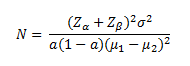In the above equation, µ1- µ2  is the expected difference between two means and σ2 is the common variance of the continuous variable.

Power is lowered as the proportion of the population (a) with an attribute becomes further away from 0.5, which in turn indicates that the proposed sample size will only be sufficient to detect larger effects sizes as the prevalence of the attribute decreases. In general, effect sizes that can be detected in this study are small to moderate (if the whole study size is used) and moderate to large (if a subgroup analysis is performed).

Below we present a graph showing the power of a two-group comparison of a continuous measure as a function of the effect size [(µ1- µ2)/σ] and a common sample size in the two groups.

Comparison of Two Group Means

As a Function of Effect Size and the Sample Size

α = 0.05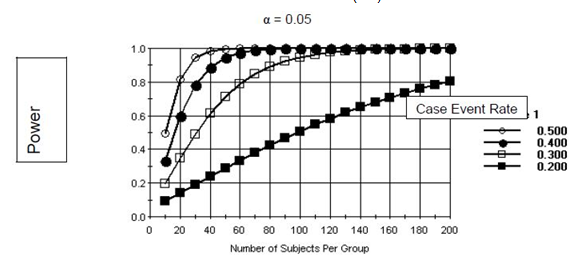As can be seen from the graph, if substantial effect sizes can be hypothesized, the number of required samples for a study will be small. For instance, if the effect size is one half of a standard deviation, a sample size of at least 80 per group will be sufficient to detect this effect when testing at the a= 0.05 level. To have adequate power to detect smaller effects will require larger sample sizes. It is likely that most effects sizes being proposed in the LTRC will fall into the range presented in the above figure.

Analysis of Proportions

Study size calculations for analyses involving proportions are functions of the overall study size, the proportion of participants in each of the comparison groups and the difference between the expected proportion of events in the different groups (9). A study size calculation for a two-group comparison of proportions has the following formula: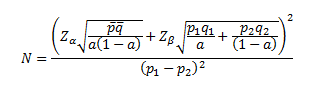In the above equation, p1 is the probability that someone with the characteristic will have a particular event, p2 is the probability that someone without the characteristic will have the event,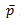is the weighted average (weighted by a) of p1 and p2, and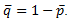.

p~ Studies to compare two groups that include most participants (for example N=1600 and “a” near 0.5) can have low values of "a" (e.g., 0.1) and low probabilities of outcomes (e.g., 0.1) and still have adequate power to detect relative risks of 2 (93%). However, if a subgroup analysis of 300 participants is performed (such as might be the case if two groups were being compared that only differed with respect to the timing of disease progression), both "a" and the probability of and outcome would have to be higher (e.g., "a" = 0.3, probability of an outcome = 0.2, power = 0.94 to detect a relative risk of 2.0).

Below we have presented two figures showing the power to detect specified alternatives of p1 and p2 assuming that equal numbers of specimens are used in each group, and the proportion of specimens with an attribute (the control event rate) is low (0.1) or moderate (0.3). In both of these figures, we have assumed that the proportion of case specimens with the attribute (the case event rate) will be higher in the alternative. As can be seen from these figures, small sample sizes will be sufficient to detect large differences between event rates. If smaller differences between the two event rates are postulated in the alternative hypothesis, larger study sizes will be necessary.

Comparison of Two Proportions

Control Event Rate Low (0.1)Comparison of Two Proportions

Control Event Rate Moderate (0.3)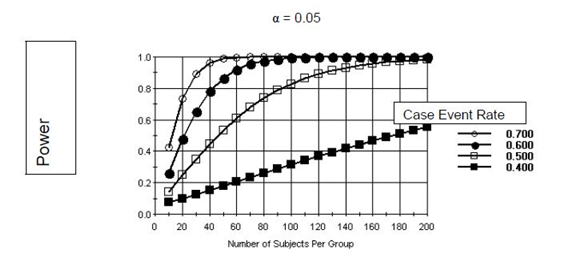Missing Data and Compliance

The DCC will adjust study size requirements using the methods of Lachin (8). Under an assumption that data are missing at random one divides the complete data study size by the estimated proportion of individuals expected to have complete studies to arrive at the final study size estimate.

Regression Models

Multivariate regression models allow one to compare the statistical strength of associations among several risk factors in the presence of markers and co-factors. Power is usually increased when using regression models compared to simple univariate comparisons. For continuous endpoints, inclusion of important independent variables in the regression equation serves to reduce the error variance for all other comparisons. For logistic regression, there is also a bias in estimation of the odds ratio, but the direction of the bias can be positive or negative. Thus, regression models are important because they increase the efficiency of proposed comparisons. However, it is required to insure that there are sufficient numbers of participants to allow regression analyses to take place. LTRC investigators will use the convention of having at least 10 observations for each planned regressor (independent variable) in a multivariate analysis to insure that the sample size is adequate for this type of analysis.

Data Analysis

## Horizontal Tabs

Overview

Data analyses will be carried out in the LTRC for two main purposes. One is to monitor CC performance and the second is to perform appropriate analyses of all study data with particular emphasis on evaluation of comparisons among participant groups. It is anticipated that recruitment and status reports will be generated on a monthly schedule and monitoring reports providing more detailed information about data quality and performance of the CCs and CLs will be generated on quarterly performance reports that will be presented to the SC, the NHLBI and the OSMB. Analyses of study specific objectives will be performed on an "as needed basis." This will include support, as directed by the NHLBI and the OSMB, for discrete and collaborative studies between the LTRC and other investigators.

Analyses for the Studies

Primary analyses for each study will focus on estimating group differences for the designated primary end point and developing statistical models to determine associations and relationships between dependent variables and risk factors. Particular analysis methods will depend on the type of study being performed and the type of end point and covariates being collected.

It will be essential to adjust study results for potential confounding factors. Three general approaches are proposed: matching, stratification, and regression analyses. In observational studies the group divisions can lead to different profiles of known risk factors in the groups because the group divisions are not randomized. This creates problems when trying to determine which of the risk factors are responsible for a particular outcome. Matching and stratification represent a non-parametric way to adjust for potential confounding variables while regression models represent a parametric method. Matching and stratification are used to "adjust out" confounding effects when one is not interested in estimating those effects and regression models are used when one is interested in comparing the effects due to a collection of risk factors. Both of these techniques are important when performing outcome analyses. Not only do regression models control for potential confounding from markers and cofactors, they can also be used to determine if effect modifiers are present which accelerate or delay cellular and biochemical changes involved in the progression of a lung disease. These effect modifiers are usually included in regression models in the form of interactions.

Since there are no plans to follow participants after they are enrolled into the study, it will be necessary to construct disease progression profiles from the individual data points that have been collected. Regression methods will be used to accomplish this aim. As part of the data collection efforts, the LTRC investigators will be asked to determine (or estimate) the amount of time that has transpired since the participant first developed the conditions that lead to the disease. It is expected that these estimates will be crude, but it may be possible to at least postulate that the disease is in its early stages, middle stages, or late stages.

If it is possible to actually estimate an "age" at which the disease process was initiated, the time since initiation of the disease is the difference between age of the participant at the time of the visit and the age at which the disease was initially developed. A time measure such as this can be used as a blocking factor in regression analyses, or as an actual regressor (independent variable). The identification of such a variable would allow the LTRC investigators to compare the cellular and biochemical progression of the different lung diseases in a very meaningful way. Under this scenario, it would be possible to develop disease type by time interactions to compare and contrast the different cellular and biochemical changes that take place between the different lung diseases being studied in the LTRC.

If it is only possible to create definitions for early, middle, and late stages, it will be more difficult to compare the disease processes of the different lung diseases since it will not be possible to determine if "early," "middle", and "late" mean the same thing in each disease. In this case, the "timing" variable would either not make sense or would be difficult to interpret when included in interaction analyses. However, the ordinal nature of the determinations would allow meaningful comparisons within a specific disease, and one could track the cellular and biochemical changes that occur within a lung disease type.

The DCC will develop regression and analysis of variance models that will provide meaningful interpretations of the LTRC data. It is anticipated that standard analysis methods would be used for continuous variables and for categorical variables, logistic regression would be used. For linear regression, tests will be made to determine the goodness of fit by examining the residuals of the analysis using influence analysis and the Hosmer-Lemeshow test (14) will be used to test residuals.

Missing Data

Some missing data are anticipated. If data are missing at random, there will be a loss in efficiency of the proposed analyses, but bias will not be introduced into the study by not accounting for the missing data. We have assumed that we will have outcome information on 85% follow-up on LTRC participants. Per Appendix C this is at least 1300 participants through 2010 and 2700 through 2014. A review of power curves and tables for sample numbers up to 1600 participants, suggests that, there will be sufficient power to address the study objectives, even when the sample of evaluable participants is lower than 1000.

However, if data are not missing at random, there could be a bias in some of the estimation and inference routines used in this study. We will use the multiple imputation procedure developed by Rubin to correct for this type of bias (15).

Conclusions

A preliminary power analysis has been performed for the LTRC. It has been found that the proposed number of participants (N = approximately 1600) is sufficient to allow for small to moderate effects to be detected with adequate power (at least 80%) when testing at the alpha level of 0.05 (two-sided tests) and for larger effect sizes if subgroup analyses are to be performed.

The LTRC offers a unique opportunity to incorporate variables depicting cellular morphology differences, lung morphology differences, lung tissue biochemical differences and genetic differences of different diseases to show how these variables impact the progression of the various lung diseases. The models presented here are general and robust enough to allow important research to be done in this area.